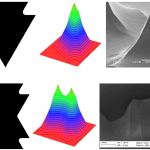# Multiplexed Needles

Current Collaborators: Professor A. I. Akinwande (EECS), B. Gassend (Ph.D.’07)
Funding: DARPA/MTO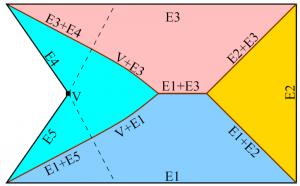Fig 1. An etch mask with five straight edges (E1 through E5) that has a concave boundary defined by the straight edges E4 and E5, which meet at vertex V. The silicon below the mask is etched with an unpassivated DRIE step. The regions determined by concave stretches of boundary are shown, as well as the ridges that will result from the interaction of the different mask edges. The dotted lines delimit the area that is controlled by the vertex V, the boundaries of which are parabolic.

Needles are widely used in a broad range of applications including drug delivery, field enhancement, and packaging (anchoring of devices). In all these applications, scaling-down of the needles is advantageous in ways that are intimately related to their function. For example, scaled-down needles for biological probing and drug delivery have a three-dimensional geometry designed to decrease the trauma to the skin and tissue due to penetration, while still providing enough stiffness to avoid structural failure of the needle during insertion. Also, scaled-down needles allow local probing of a tissue, and a massive array of needles would enable global monitoring of the biological system at length scales far larger than the needle dimensions. Similarly, scaled-down needles used as electrospray emitters are hundreds of microns tall, hundreds of microns apart, and have submicron tip radii so that they can interact with liquids and turn-on with low voltage. Finally, the needles used as electron field emitters have nanometer-sharp tips to achieve low-voltage operation.

Model for Needle Design: We have concentrated our research on the development of silicon-based Deep Reactive Ion Etched (DRIE) needle-like solid structures, i.e., DRIE-patterned high aspect-ratio solid structures that have sidewalls with non-constant slope along their heights and that end in sharp protrusions. In particular, we have developed a model that is very helpful to design complex high aspect-ratio silicon needles. In this model, the needle-like structures are fabricated using a sequence of passivated (the standard DRIE process) and unpassivated (no fluorocarbon-based plasma in the process) DRIE steps that in combination with an etch mask achieves a certain geometry, including ultrasharp tips. The model does not simulate the dynamics of the interaction between the plasma and the substrate, or makes corrections for microloading, scalloping, etch window variation, or any other effect that could affect the etch uniformity. Each etching step is described by the etch depth and etch profile:

•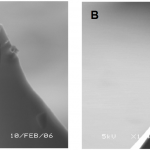Fig. 2. A sharp silicon tip formed when three etch fronts meet (A), and a blade-like tip formed when four etch fronts mee

Each unpassivated DRIE step is modeled as an etch process with an etch rate that is a function only of the normal of the surface being etched. If this function is uniform, the resulting etch is isotropic. However, the bias power of the unpassivated DRIE step produces some anisotropy, making the vertical etch rate higher than horizontal etch rate.

• or the passivated DRIE steps, the etch proceeds vertically at a uniform rate, except in areas directly below the etch mask, which are assumed unetched by the plasma. This model for the passivated DRIE implies that the bottom of a partially etched wafer remains flat across the full extent of the etch surface.

The resulting shape after a passivated or unpassivated DRIE step is complete can be represented by two 2D functions that vary along the surface of the wafer. In both cases, a first function z(x,y) defines the depth of the silicon etch at the beginning of the step at each (x,y) location, where x, and y are Cartesian coordinates on the wafer surface using a coordinate system with axes in the <100> direction (for example, the x- and y- axis can be respectively parallel and perpendicular to the wafer flat in a <100> wafer). For passivated DRIE steps, a second function M(x,y) defines the areas of the wafer that are protected by an etch mask. The function M(x,y) only takes on the values 1 or 0 indicating the presence or absence of masking material, respectively. A passivated DRIE step does not produce undercut, i.e., the etch mask acts as an umbrella that protects the silicon directly below it, even if it is not in direct contact to the etch mask. Therefore, after a passivated DRIE step of depth d, the function z becomes z’

z′ = z (x,y) + d · M (x,y)

(1)

For unpassivated DRIE steps, a concave shape function  is used instead as second function. S can take on real values or −∞, and for normalization purposes . In general, the shape function S is smooth wherever it is finite. After an unpassivated DRIE step of depth d, the function z becomes z’

(2)

The maximization considers all the positions where the silicon is exposed (i.e., it includes silicon not directly in contact with the etch mask) to determine how deep the etch has progressed at a given (x,y) point. In effect, the shape function S defines the cavity that a hard mask with a point-hole in it creates during an unpassivated DRIE step, once the silicon directly below the hole has receded by a unit distance. The model of the unpassivated DRIE step states that the etching proceeds from the exposed silicon surface in all directions, with an etchrate that is a function of the tilt of the sidewall by the shape function S. From fabrication results we concluded that S defines a roughly ellipsoidal shape with a vertical z-axis length twice the length of any of the in-plane x and y axes. Also, the model can take into account a slight lattice orientation-dependent anisotropy in the silicon etch that was experimentally observed. Specifically, the fabrication results suggest that unpassivated DRIE steps have a small dependence in crystal plane orientation that causes a slight bulge in the x and y axes of an ellipsoidal etch, compared to the x and y axes rotated by 45° with respect to <100> (see Section III). In the model, the shape function S for unpassivated DRIE steps carried out on a <100> wafer is

(3)

where β is a parameter that describes the directionality of the etch, and a is a parameter that characterizes the lattice orientation-dependent anisotropy. When the result of Eq. 3 is not a real number, it is set to S(x, y) = −∞. The constant β was empirically found to vary across the wafer with a typical value around 2. Taking from now on β=2, empirical results lead to values of α of about 18.5.  For α = 0, Eq. 3 results in an ellipsoid with a z-axis of length = 1, and x- and y-axis of length of 1/β, i.e., 0.5. The x2y2 term in Eq. 3 is the lowest-order correction of the symmetry of the etch shape. This correction was sufficient to explain the small anisotropies that were found in the experimental results on <100> Si wafers.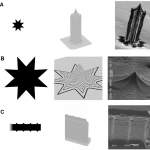Fig. 4. Mask (left), graphical prediction of model (center), and fabrication result (right) of a pencil-like needle (A), a conic needle (B), and a needle with a serrated top edge (C).

Ridge Formation and Ultrasharp Tips: It is possible to create ridges in an otherwise smooth surface of needle-like structures etched with a single unpassivated DRIE step. These discontinuities are predicted by the model. According to the model, the ridges occur when control of the etch depth jumps discontinuously from one point to another on the edge of the mask, i.e., where two or more etching fronts meet. As illustrated in Fig. 1, the etch depth of most points below the mask is set by a single point on the edge of the mask. In the figure, each colored region corresponds to points whose depth is set by a given concave stretch of the mask boundary (a stretch in which the border only turns away from the inside of the mask). In each region, the maximum in Eq. 2 is reached at exactly one point on the corresponding concave stretch of the boundary. When two regions meet, the maximum is attained in more than one way. In this case, moving slightly away from the boundary causes different mask edges to dominate. The regions dominated by two distant mask edges will not have the same surface normal; therefore, a normal discontinuity is present where these two regions intersect. Likewise, when three regions intersect, a tip is formed.

There are two ideas from computational geometry that are helpful to determine the location of the ridges. These relationships are only exact if α is zero, i.e., when S(x, y) has symmetry of revolution. However, the fabrication results demonstrate that the departure from symmetry of revolution in the unpassivated DRIE steps is small enough that it does not have a significant impact on the final shape of the structure. The two ideas are the Medial Axis Transform (MAT) and the distance transform. Specifically:

• The MAT of a region is the set of points for which there is more than one closest point on the boundary of the region. For a polygon, the MAT is made up of portions of angular bisectors of edges, perpendicular bisectors of vertices, and parabolas that have one edge as a directrix and one vertex as a focus. The MAT of the etch mask defines the projection of the ridges on the etch mask surface. The MAT can easily be sketched by hand and is an excellent aid in designing mask shapes for microneedles.
• The distance transform of a mask shape is a function DT(x,y) that maps each point in the plane to the distance from that point to the closest point outside the mask. This function is zero in areas not covered by the mask, and steadily increases inside the mask. Eq. 2 is closely related to the definition of the distance transform, so that the final shape of the needle-like structure can be expressed by

(4)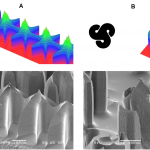Fig. 5. Mask (top left), graphical model prediction (top right), and fabrication result (bottom) for tightly packed row of star-shaped needles (A) and a swirl needle (B).

where f is a function that depends on the sequence of etches that was performed. The ridges arise because DT(x, y) is not smooth on the MAT.

So far, only needle-like structures formed from a single unpassivated DRIE step have been discussed. Another type of ridge appears when a passivated DRIE step is part of the sequence because the passivated DRIE steps introduce discontinuities in f. These discontinuities lead to discontinuities in z(x,y), with an edge at the top of the corresponding vertical DRIE sidewalls.

Fabrication results show that DRIE-patterned sharp tips can only be reliably obtained if the tip is formed at the meeting point of only three ridges (Fig. 2 A). If one of the ridges is shifted because the etch is progressing at a different rate compared to the other two ridges, the position of the tip is moved slightly, but the tip remains sharp. This is unlike the case where four or more ridges are involved in the formation of the tip, in which the tip can become a straight edge (Fig. 2 B). Moreover, the three ridges should ideally meet at 120° from each other so that the tip is symmetric.

Model Comparison with Fabricated Structures: This section provides only a qualitative evaluation of the model, that is, an evaluation of the visual resemblance of the etched structures to the predictions of the model. Because of the large radial non-uniformity typically attained with DRIE, a quantitative comparison would require the use of etch depths and shape functions that depend on the position on the wafer. All experimental results in this section come from <100> silicon wafers.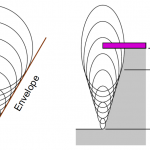Fig. 6. The envelope of a set of evenly spaced circles defines a straight line (left). Therefore, a set of tightly spaced passivated and unpassivated DRIE steps defines an etch profile with constant slope, except at the top of the profile.